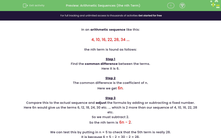Comprehensive & curriculum aligned

In this worksheet, students add together two numbers - both of which have three digits. They use a formal written/column method.Key stage:  KS 2

Curriculum topic:   Number: Addition and Subtraction

Curriculum subtopic:   Add/Subtract up to Four-Digit Numbers

Difficulty level:Worksheet Overview

This worksheet helps children to learn about the addition of three digits number.

For example -

Question - Add the numbers together.

 + 1 5 8 9 6 1